#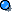Concepts from Movie

Rotational Inertia I=mr2

Angular Momentum

Frequency

Concepts from Book

(click on thumbnails to enlarge, us your 'back' button or arrow to return)

Rotation vs Revolution

Linear Speed vs Rotational Speed(or angular speed)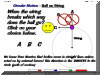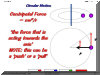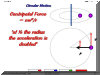Linear Speed AnimationPowerPoint - requires Internet Explorer or PowerPoint Viewer to view)

Simulated Gravity

Concepts on Chapter 9 Take Home 2

Forces causing circular motion

Simulated Gravity

Centripetal Force

Linear Speed and Circular Motion

Relationship between radius and linear speed

Relationship between pseudo force (centrifugal)

Rotational Motion

Circular motion Videos

Refer to these websites for more explanations

 Uniform Circular MotionUniform circular motion can be described as the motion of an object in a circle at a constant speed. As an object moves in a circle, it is constantly changing its direction. In all instances, the object is moving tangent to the circle. Since the direction of the velocity vector is the same as the direction of the object's motion, the velocity vector is directed tangent to the circle as well. The animation below depicts this by means of a vector arrow.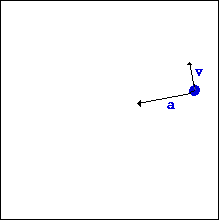An object moving in a circle is accelerating. Accelerating objects are objects which are changing their velocity - either the speed (i.e., magnitude of the velocity vector) or the direction. An object undergoing uniform circular motion is moving with constant speed; nonetheless, it is accelerating due to its change in direction. The direction of the acceleration is inwards. The animation at the right depicts this by means of a vector arrow. The final motion characteristic for an object undergoing uniform circular motion is the net force. The net force acting upon such an object is directed towards the center of the circle; it is said to be an inward or centripetal force. Without such an inward force, an object would continue in a straight line, never deviating its direction. Yet, with the inward net force directed perpendicular to the velocity vector, the object is always changing its direction and undergoing an inward acceleration. CENTRIPETAL FORCE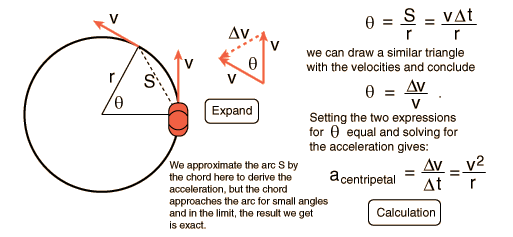Centripetal force is the force that is applied in the direction of the rotation axis that makes the object move in a circular path. CENTRIFUGAL FORCE Centrifugal force is the 'pseudo' or 'effective' force that is used to  explain the behavior of objects from a frame of reference which is rotating.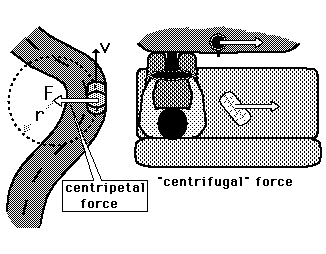The driver of a car on a curve is in a rotating reference frame and he could invoke a "centrifugal" force to explain why his coffee cup and the carton of eggs he has on the seat beside him tend to slide sideways. The friction of the seat or dashboard may not be sufficient to accelerate these objects in the curved path. The centrifugal force is a useful concept when the most convenient reference frame is one which is moving in a curved path, and therefore experiencing a centripetal acceleration. Since the car above will be experiencing a centripetal acceleration v2/r, then an object of mass m on the seat will require a force mv2/r toward the center of the circle to stay at the same spot on the seat. From the reference frame of a person in the car, there seems to be an outward centrifugal force mv2/r acting to move the mass radially outward. In practical descriptive terms, you would say that your carton of eggs is more likely to slide outward if you have a higher speed around the curve (the velocity squared factor) and more likely to slide outward if you go around a sharper curve ( the inverse dependence upon r).

SAMPLE QUESTIONS

A. Suppose two identical objects go around in horizontal circles of identical diameter but one object goes around the circle twice as fast as the other. The force required to keep the faster object on the circular path is

1. the same as

2. one fourth of

3. half of

4. twice

5. four times

the force required to keep the slower object on the path.

B. Suppose two identical objects go around in horizontal circles with the same speed. The diameter of one circle is half of the diameter of the other. The force required to keep the object on the smaller circular path is

1. the same as

2. one fourth of

3. half of

4. twice

5. four times

the force required to keep the object on the larger path.

C. A turntable rotates a speed of 1 revolution/sec. A gentleman bug sits half way between a ladybug and the center of the turntable. The angular speed of the gentleman bug is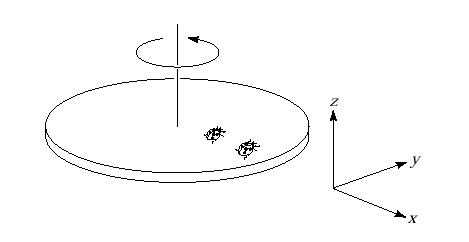1. the same as

2. one fourth of

3. half of

4. twice

5. four times

the angular speed of the ladybug.

E. A woman rides in the amusement park ride called the "ROTOR". Which of the free-body force diagrams best represents the situation?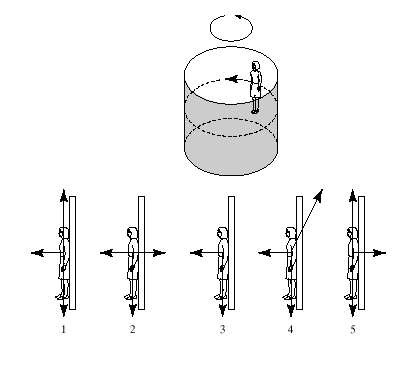A. The answer is 5. As the velocity increases the centripetal force required to maintain the circle increases as the square of the speed.

B. The answer is 4. The centripetal force needed to maintain the circular motion of a object is inversely proportional to the radius of the circle. Everybody knows that it is harder to navigate a sharp turn than a wide turn. Now you know why.

C. The answer is 1. Angular speed is the rate at which the angle changes. Both bugs move through 2p radians in 1 sec.

D. The answer is 4. The ladybug needs to cover twice as much distance along the circumference of her circle than the gentleman bug does. As the both make a complete circle in one second the tangential speed must be twice as large for the ladybug.

back to questions

E. The answer is 1. The woman is subject to three forces: her weight, the normal force, and a frictional force. The normal force provides the centripetal acceleration to maintain her circular motion and the frictional force keeps her from sliding down the wall. As the angular speed of the Rotor increases the normal force must increase to provide the needed acceleration to maintain the woman in her circular path. The increasing normal force increases the maximum possible static friction force.

back to questions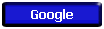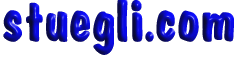Copyright © 2005 -  S. B. EglI